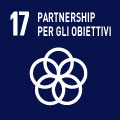# 73513 - NUMERICAL METHODS M

### SDGs

L'insegnamento contribuisce al perseguimento degli Obiettivi di Sviluppo Sostenibile dell'Agenda 2030 dell'ONU.## Conoscenze e abilità da conseguire

A successful learner from this course will be able to: a) deal with numerical analysis topics such as: accuracy, truncation and round-off errors, condition numbers, convergence, stability, curve-fitting, interpolation, numerical differentiation and integration, numerical linear algebra; b) deal with numerical methods for solving ordinary and partial differential equations, with finite difference and finite element methods for parabolic and elliptic partial differential equations.

## Contenuti

• The course will cover fundamental topics in numerical computation, including in particular:

- Computer arithmetic;

- Solving nonlinear equations;

- Solving systems of linear and nonlinear equations;

- Least squares approximation and data filling;

- Polynomial and spline interpolation.

• Throughout the course, lectures will be supported by computer lab activities, during which the Matlab software will be used for experimenting with numerical phenomena and solving practical problems.

## Testi/Bibliografia

Learning material will be provided during the course in the form of slides, exercises and quizzes and will be dowloadable from the IOL platform.

- U. Ascher, C. Greif, A first course in Numerical Methods, SIAM, 2011.

- A. Quarteroni, R. Sacco and F. Saleri, "Numerical Mathematics, Second Ed.", Springer, 2007.

- M.T. Heath, "Scientific Computing. An introductory survey", McGraw-Hill, 1997.

## Metodi didattici

The course involves theoretical lectures and practical work based on computational tools such as Matlab.

Quizzes and solved exercises will be provided as supplementary material.

Attendance at lectures and computer lab sessions is of fundamental importance in order to learn the contents of the course.

## Modalità di verifica dell'apprendimento

Assessment is based on a written exam including exercises with Matlab and written questions.

Sample tests will be provided to help students assess their level of learning.

## Strumenti a supporto della didattica

Lectures, lecture slides, exercises, quizzes, computer lab activities with Matlab.

## Orario di ricevimento

Consulta il sito web di Carolina Vittoria Beccari